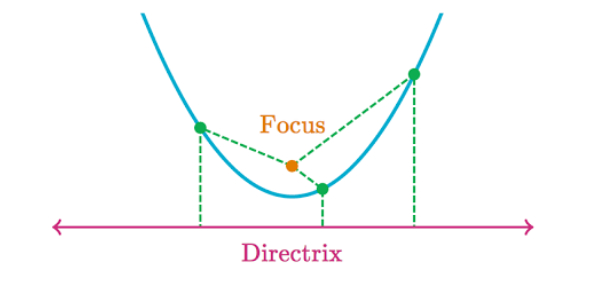# Parabola Quiz 10.4

Approved & Edited by ProProfs Editorial Team
The editorial team at ProProfs Quizzes consists of a select group of subject experts, trivia writers, and quiz masters who have authored over 10,000 quizzes taken by more than 100 million users. This team includes our in-house seasoned quiz moderators and subject matter experts. Our editorial experts, spread across the world, are rigorously trained using our comprehensive guidelines to ensure that you receive the highest quality quizzes.
| By Sevans1014
S
Sevans1014
Community Contributor
Quizzes Created: 2 | Total Attempts: 2,233
Questions: 8 | Attempts: 1,942SettingsQuiz on the beginning of Chapter 10 in Integrated Mathematics.

• 1.

### What is the equation of the parabola that is produced by translating the graph of y = x2 three units to the left?

• A.

Y = (x+3)^2

• B.

Y = x^2 - 3

A. Y = (x+3)^2
Explanation
The equation of the parabola that is produced by translating the graph of y = x^2 three units to the left is y = (x+3)^2. This is because when we translate a graph to the left, we subtract the amount of units we want to translate from the x-coordinate. In this case, we subtract 3 from x, resulting in (x+3). The rest of the equation remains the same, so we have y = (x+3)^2.

Rate this question:

• 2.

### For the graph of y = x^2 + 7, what is the equation of the line of symmetry?

• A.

X = 7

• B.

X = 0

B. X = 0
Explanation
The equation of the line of symmetry for a parabola in the form y = ax^2 + bx + c is given by x = -b/2a. In this case, the equation y = x^2 + 7 is already in the standard form, so the coefficient b is 0. Therefore, the line of symmetry is x = -0/2(1) = 0.

Rate this question:

• 3.

### For the graph of y = x^2 - 5, what are the coordinates of the vertex?

• A.

(0, -5)

• B.

(-5, 0)

A. (0, -5)
Explanation
The vertex of a parabola in the form y = ax^2 + bx + c is given by the coordinates (-b/2a, f(-b/2a)). In this case, the equation is y = x^2 - 5, so a = 1, b = 0, and c = -5. Plugging these values into the formula, we get (-0/2(1), f(0/2(1))) = (0, f(0)) = (0, -5). Therefore, the coordinates of the vertex are (0, -5).

Rate this question:

• 4.

### Describe the graph of y = -(x - 2)^2.

• A.

Translate y = -x^2 two units to the right

• B.

Translate y = -x^2 two units down

A. Translate y = -x^2 two units to the right
Explanation
The given equation, y = -(x - 2)^2, is a translation of the graph of y = -x^2 two units to the right. This means that every point on the graph of y = -x^2 is shifted horizontally by two units to the right to obtain the graph of y = -(x - 2)^2. The negative sign in front of the equation indicates that the graph is reflected across the x-axis. The vertex of the graph is at (2, 0), which is two units to the right of the vertex of y = -x^2.

Rate this question:

• 5.

### What are the x-intercepts for the graph of y = (x+2)(2x-3)?

• A.

X = 2 and x = -3

• B.

X = -2 and x = 3

• C.

X = 3/2 and x = -2

C. X = 3/2 and x = -2
Explanation
The x-intercepts of a graph are the points where the graph intersects the x-axis. In order to find the x-intercepts, we set y equal to zero and solve for x. In the given equation y = (x+2)(2x-3), we set y = 0 and solve for x. By factoring the equation, we get (x+2)(2x-3) = 0. Setting each factor equal to zero, we find x = -2 and x = 3/2. Therefore, the x-intercepts for the graph of y = (x+2)(2x-3) are x = 3/2 and x = -2.

Rate this question:

• 6.

### For the equation, y = (x + 1)(x - 3), what is the value of y when x = 1?

• A.

-1

• B.

-4

B. -4
Explanation
When x = 1, we can substitute this value into the equation y = (x + 1)(x - 3). By substituting x = 1, we get y = (1 + 1)(1 - 3) = (2)(-2) = -4. Therefore, the value of y when x = 1 is -4.

Rate this question:

• 7.

### Simplify:   5a3(-2a)

• A.

3a^3

• B.

-10a^4

B. -10a^4
Explanation
The given expression can be simplified by multiplying the coefficients and adding the exponents of the variable. In this case, the coefficient 5 and -2 multiply to give -10, and the variable "a" has an exponent of 3 in the first term and 1 in the second term. Therefore, the simplified expression is -10a^4.

Rate this question:

• 8.

### Simplify:    (-4x5y)4

• A.

256x^20y^4

• B.

256x^9y^4

A. 256x^20y^4
Explanation
To simplify the expression (-4x5y)4, we need to apply the exponent to each term inside the parentheses. The exponent 4 is distributed to both -4, x, 5, and y. Since (-4)^4 is equal to 256, x^5 raised to the power of 4 becomes x^20, and y^4 remains the same, the simplified expression is 256x^20y^4.

Rate this question:

Related TopicsBack to top